SYLLABUS  Previous: 4.3.1 Transformation to log-normal  Up: 4.3 Methods for European  Next: 4.3.3 Black-Scholes formula

4.3.2 Solution of the normalized diffusion equation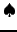[ SLIDE Fourier-Laplace fwd - bck || Solution binary options || same VIDEO as previous section: modem - LAN - DSL]

Readers interested in solving the diffusion equation (4.3.1#eq.5) analytically are likely to be familiar with the Fourier-Laplace transform that is commonly used to solve initial and boundary value problems. Others may skip the whole derivation and verify that the final result (4.3.2#eq.11) indeed satisfies the Black-Scholes equation (3.4#eq.4).
Start by transforming (4.3.1#eq.5) with a Laplace transform in time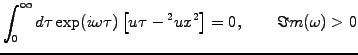(4.3.2#eq.1)

Note that the condition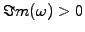is important to ensure causality. Integrate the first term by parts and substitute a Dirac function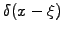for the initial condition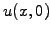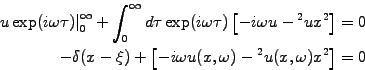(4.3.2#eq.2)

The notation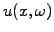refers to the Laplace transform of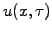. Spatial derivatives are dealt with a Fourier transform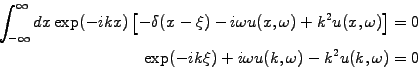(4.3.2#eq.3)

and yields an explicit solution in Fourier-Laplace space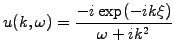(4.3.2#eq.4)

The pole in the complex plane for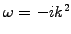needs to be taken into account when inverting the Laplace transform in a causal manner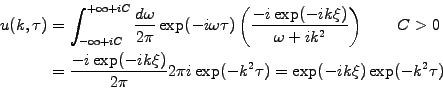(4.3.2#eq.5)

where the residue theorem has been used to evaluate the complex integral, closing the contour in the lower half plane where the phase factor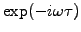decays exponentially. Invert the Fourier transformation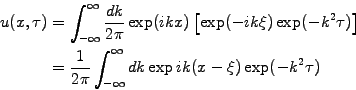(4.3.2#eq.6)

and use the formula (3.323.2) from Gradshteyn & Ryzhik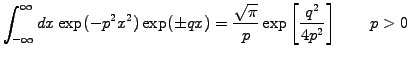(4.3.2#eq.7)

here with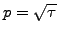and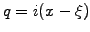to write down a solution of the diffusion equation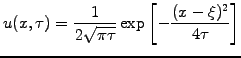(4.3.2#eq.8)

This shows that a Dirac functionassumed as initial condition in (4.3.2#eq.2) spreads out into a Gaussian as time evolves forward. A superposition of a whole series of Dirac functions can now be used to decompose any arbitrary initial condition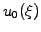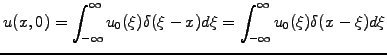(4.3.2#eq.9)

and after evolving each Dirac functions separately using (4.3.2#eq.8), can again be superposed at a later time when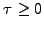: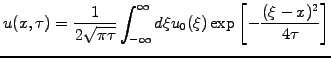(4.3.2#eq.10)

Transforming back into financial variables (4.3.1#eq.1), some algebra finally yields a general formula for the price of a binary option with a terminal payoff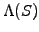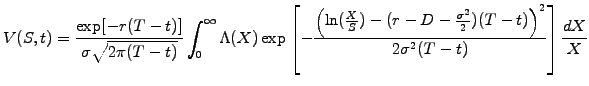(4.3.2#eq.11)

SYLLABUS  Previous: 4.3.1 Transformation to log-normal  Up: 4.3 Methods for European  Next: 4.3.3 Black-Scholes formula